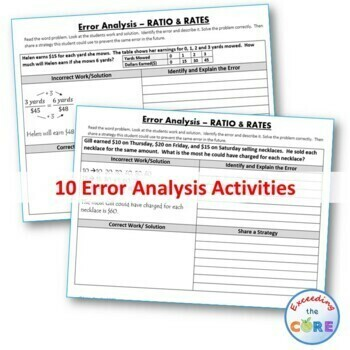# RATIOS AND RATES Word Problems - Error Analysis (Find the Error)Subject
Resource Type
File Type

PDF

(1 MB|14 pages)
Standards
Also included in:
1. - Great for online learning and distance learning!Have your students apply their understanding of math concepts with these ERROR ANALYSIS activities. This GROWING BUNDLE resource includes 8 sets (a total of 80 questions) of Common Core WORD PROBLEMS that are solved incorrectly. Students have to i
\$24.00
\$19.20
Save \$4.80
2. - Great for online learning and distance learning!This middle school ERROR ANALYSIS BUNDLE includes 230 real-world word problems (23 sets) that are solved incorrectly for grades 6-8. Students have to IDENTIFY THE ERROR, provide the CORRECT SOLUTION and share a helpful STRATEGY for solving the proble
\$69.00
\$48.30
Save \$20.70
3. - Great for online learning and distance learning!RATIOS AND RATES BUNDLE - Task Cards, Error Analysis, Word Problems with Graphic Organizers, Homework Practice Worksheet, Doodle NotesThis UNIT RESOURCE BUNDLE includes 40 task cards, 10 error analysis activities, 10 problem solving graphic organizer
\$15.00
\$11.60
Save \$3.40
• Product Description
• Standards

- Great for online learning and distance learning!

Ratios and Rates Word Problems - Error Analysis

Have your students apply their understanding of RATIOS AND RATES with these ERROR ANALYSIS activities.

Benefits of Math Error Analysis:

Giving students opportunities to identify and correct errors in presented solutions allows them to show their understanding of the mathematical concepts you have taught.

Whats Included:

This resource includes 10 real-world RATIOS AND RATES word problems that are solved incorrectly. Students have to IDENTIFY THE ERROR, provide the CORRECT SOLUTION and share a helpful STRATEGY for solving the problem. An ANSWER KEY has also been provided with examples of possible answers. Be sure to download the sample for a full overview of what you get.

How to Use:

When I present this activity to my students, I tell them that these are errors that students from my other class made and I need their help to correct them. Students LOVE correcting other students mistakes. Students can use these activities with a partner, as a warm-up , as classwork, homework, in math centers or group work.

Topics included:

✔ Factors & Multiples

✔ Ratios

✔ Unit Rate & Unit Price

✔ Equivalent Ratios

✔ Ratio Tables

✔ Rate Problems

This resource is included in my Ratios and Rates UNIT RESOURCE BUNDLE.

Common Core Aligned:

* 6.RP.1 Understand the concept of a ratio and use ratio language to describe a ratio relationship between two quantities.

* 6.RP.2 Understand the concept of a unit rate a/b associated with a ratio a:b with b ≠ 0, and use rate language in the context of a ratio relationship.

* 6.RP.3 Use ratio and rate reasoning to solve real-world and mathematical problems...

* 6.NS.4 Find the greatest common factor of two whole numbers less than or equal to 100 and the least common multiple of two whole numbers less than or equal to 12.

More RATIOS AND RATES Resources:

BUNDLE UP & Save \$\$ This resource is included in my:

If you would like to get updates on NEW and CURRENT resources...

FOLLOW me on Teachers Pay Teachers!

© Exceeding the CORE. All rights reserved. Please note - this resource is for use by one teacher only. Additional teachers must purchase their own license. Copying, editing, selling, redistributing, or posting any part of this product on the internet is strictly forbidden. Violations are subject to the penalties of the Digital Millennium Copyright Act.

Click HERE to become a Seller on TPT. { Referral by Exceeding the CORE}

Find a percent of a quantity as a rate per 100 (e.g., 30% of a quantity means 30/100 times the quantity); solve problems involving finding the whole, given a part and the percent.
Solve unit rate problems including those involving unit pricing and constant speed. For example, if it took 7 hours to mow 4 lawns, then at that rate, how many lawns could be mowed in 35 hours? At what rate were lawns being mowed?
Make tables of equivalent ratios relating quantities with whole-number measurements, find missing values in the tables, and plot the pairs of values on the coordinate plane. Use tables to compare ratios.
Understand the concept of a unit rate 𝘢/𝘣 associated with a ratio 𝘢:𝘣 with 𝘣 ≠ 0, and use rate language in the context of a ratio relationship. For example, “This recipe has a ratio of 3 cups of flour to 4 cups of sugar, so there is 3/4 cup of flour for each cup of sugar.” “We paid \$75 for 15 hamburgers, which is a rate of \$5 per hamburger.”
Understand the concept of a ratio and use ratio language to describe a ratio relationship between two quantities. For example, “The ratio of wings to beaks in the bird house at the zoo was 2:1, because for every 2 wings there was 1 beak.” “For every vote candidate A received, candidate C received nearly three votes.”
Total Pages
14 pages
Included
Teaching Duration
45 minutes
Report this Resource to TpT
Reported resources will be reviewed by our team. Report this resource to let us know if this resource violates TpT’s content guidelines.